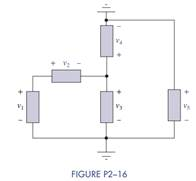Create an Account

Home / Questions / In Figure P2−16 determine which elements are in series, parallel, or neither. How many...

In Figure P2−16 determine which elements are in series, parallel, or neither. How many...

In Figure P2−16 determine which elements are in series, parallel, or neither. How many different nodes and loops are there in the circuit? Then if v2 = 3 V and v3 = 5 V, find v1, v4, and v5.Jul 28 2020 View more View LessSubscribe To Get Solution